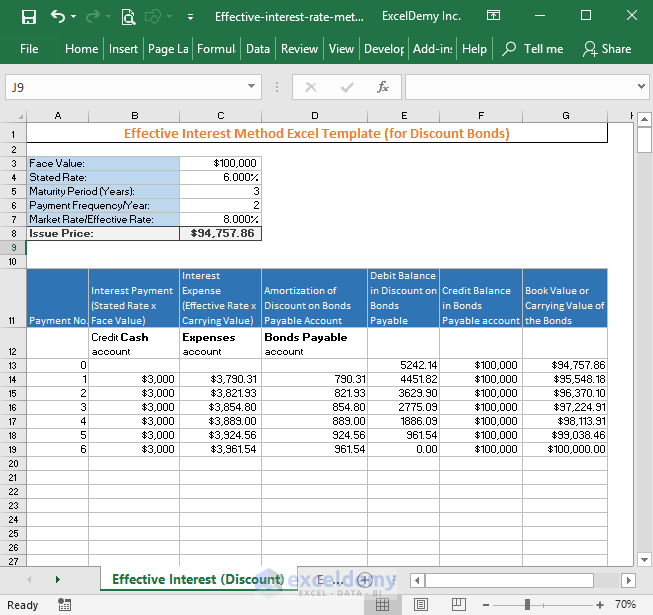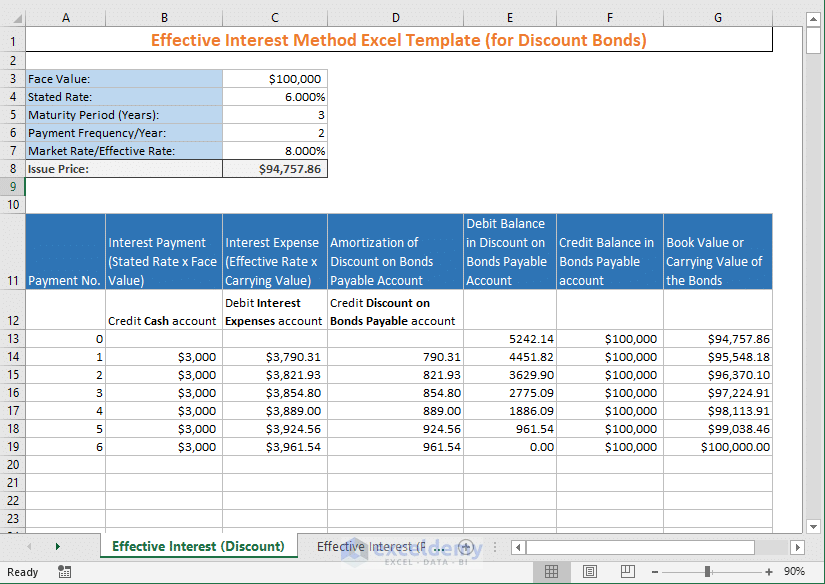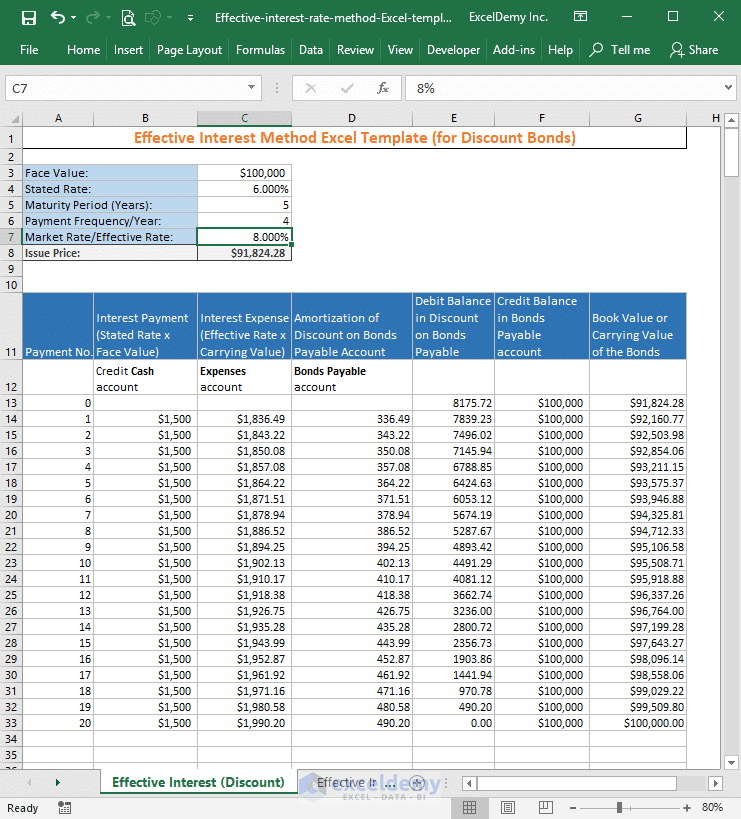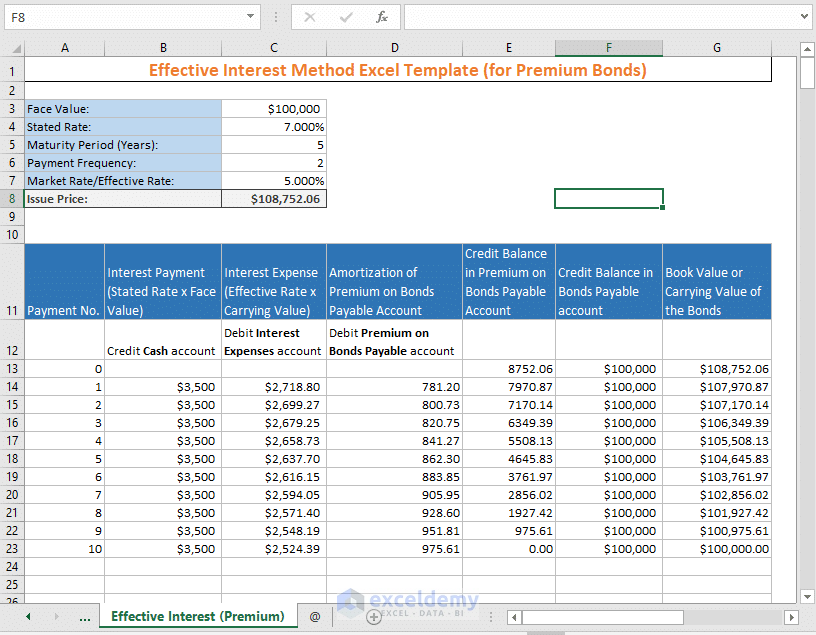# Effective Interest Rate Method Excel Template (Free)Effective Interest Rate Method Excel Template

“No Macro used. No installation is necessary. Just download and start using.” – Kawser Ahmed (Template Developer)

Created using Excel 2016 version

License: Personal Use (Not for resale or distribution)

Let us know (in the comment box) your criteria or the problems that you’re facing while using this template. We shall update the template.

It is very easy to use my developed “Effective interest rate method excel template”. Actually, you will get two templates inside the workbook (if you have downloaded the workbook).

• One template is for bonds that are sold in discount
• Another template is for bonds that are sold in premium

You will have to provide very few inputs. The inputs are:

• The face value of the bond
• Stated Interest Rate / Nominal Interest Rate
• Maturity Period of the Bonds (in Years)
• Payment Frequency of the Bond in Every Year
• Market Rate or Effective Interest Rate of the Bond

This template will output the Issue price of the Bond and show the other calculations.

## Effective interest rate method Excel template

### 1) For Discounted Bonds

Take a look at the following image. It shows the amortization table for a bond with the following details:

• Face Value: \$100,000
• Stated Rate: 6%
• Maturity Period: 3 Years
• Payment Frequency: Semi-annual
• Market Rate/Effective Interest Rate: 8%

The template shows that the Issue Price of the bond will be: \$94,757.86

And shows all the calculations for the bond maturity period.Now I will just change the maturity period from 3 years to 5 years and payments will be done quarterly.

• Face Value: \$100,000
• Stated Rate: 6%
• Maturity Period: 5 Years
• Payment Frequency: Quarterly
• Market Rate/Effective Interest Rate: 8%

Now check out the image.See how the Excel template works for bonds that are sold in premium with the following information:

• Face Value: \$100,000
• Stated Rate: 7.000%
• Maturity Period (Years): 5
• Payment Frequency: 2
• Market Rate/Effective Rate: 5.000%

Check out the following image. The template provides issue price as \$108752.06 and other calculations for the bond maturity period. And all the calculations are done automatically.If you don’t know the basic of effective interest method, how the whole thing works, then you can check out this article: Effective Interest Method of Amortization in Excel